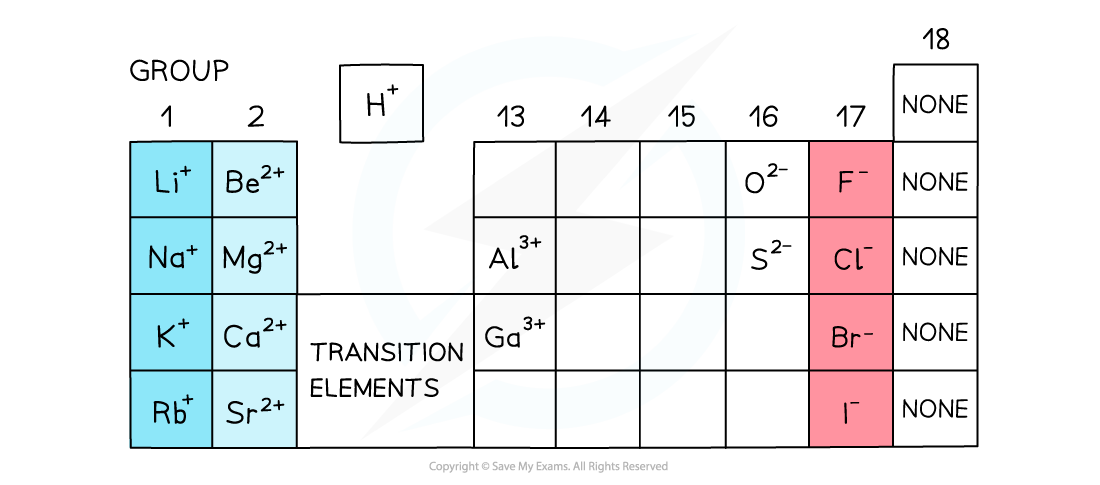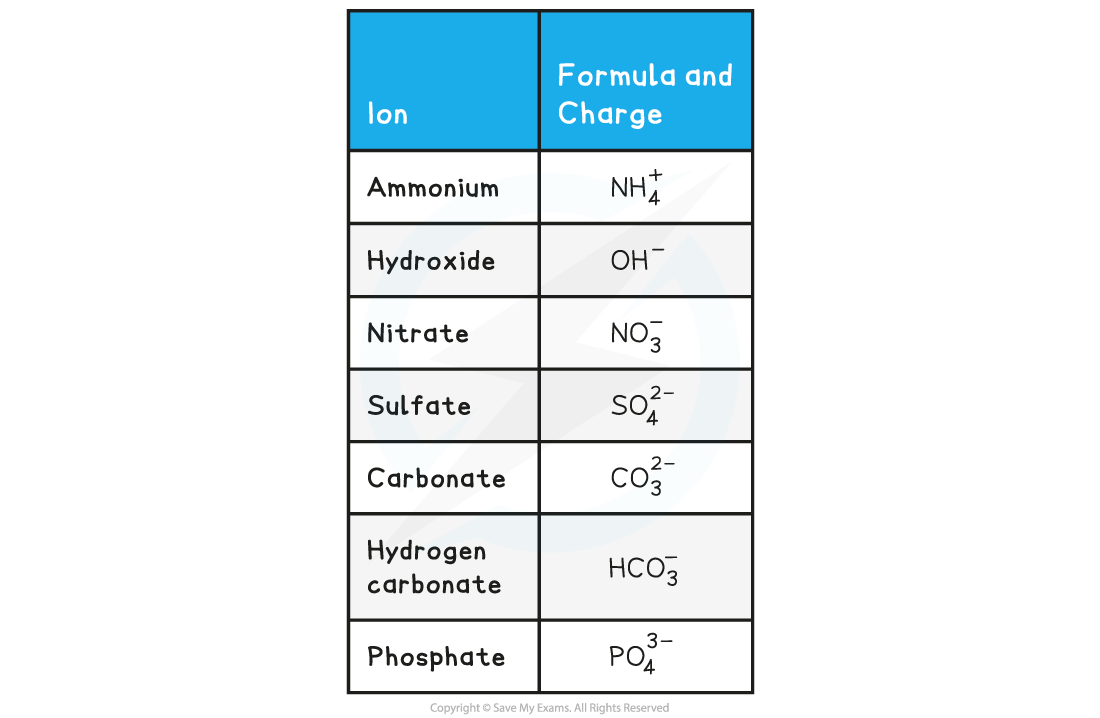# IB DP Chemistry: SL复习笔记4.1.3 Formulae & Names of Ionic Compounds

### Formulae & Names of Ionic Compounds

• Ionic compounds are formed from a metal and a nonmetal bonded together
• Ionic compounds are electrically neutral; the positive charges equal the negative charges

#### Charges on positive ions

• All metals form positive ions
• There are some non-metal positive ions such as ammonium, NH4+, and hydrogen, H+
• The metals in Group 1, Group 2 and Group 13 have a charge of 1+ and 2+ and 3+ respectively
• The charge on the ions of the transition elements can vary which is why Roman numerals are often used to indicate their charge
• This is known as Stock notation after the German chemist Alfred Stock
• Roman numerals are used in some compounds formed from transition elements to show the charge (or oxidation state) of metal ions
• Eg. in copper (II) oxide, the copper ion has a charge of 2+ whereas in copper (I) nitrate, the copper has a charge of 1+

#### Non-metal ions

• The non-metals in group 15 to 17 have a negative charge and have the suffix ‘ide
• Eg. nitride, chloride, bromide, iodide
• Elements in group 17 gain 1 electron so have a 1- charge, eg. Br-
• Elements in group 16 gain 2 electrons so have a 2- charge, eg. O2-
• Elements in group 15 gain 3 electrons so have a 3- charge, eg. N3-
• There are also more polyatomic or compound negative ions, which are negative ions made up of more than one type of atomThe charges of simple ions depend on their position in the Periodic Table

• There are seven polyatomic ions you need to know for IB Chemistry:

Formulae of Polyatomic Ions Table#### Worked Example

Determine the formulae of the following ionic compounds

1. magnesium chloride
2. aluminium oxide
3. ammonium sulfate

• Magnesium is in group 2 so has a charge of 2+
• Chlorine is in group 17 so has a charge of 1-
• Magnesium needs two chlorine atoms for each magnesium atom to be balanced so the formula is MgCl2

• Aluminum is in group 13 so the ion has a charge of 3+
• Oxygen is in group 16 so has a charge of 2-
• The charges need to be equal so 2 aluminium to 3 oxygen atoms will balance electrically, so the formula is Al2O3

• Ammonium is a polyatomic ion with a charge of 1+
• Sulfate is a polyatomic ion and has a charge of 2-
• The polyatomic ion needs to be placed in a bracket if more than 1 is needed
• The formula of ammonium nitrate is (NH4)2SO4

#### Exam Tip

Remember: polyatomic ions are ions that contain more than one type of element, such as OH-